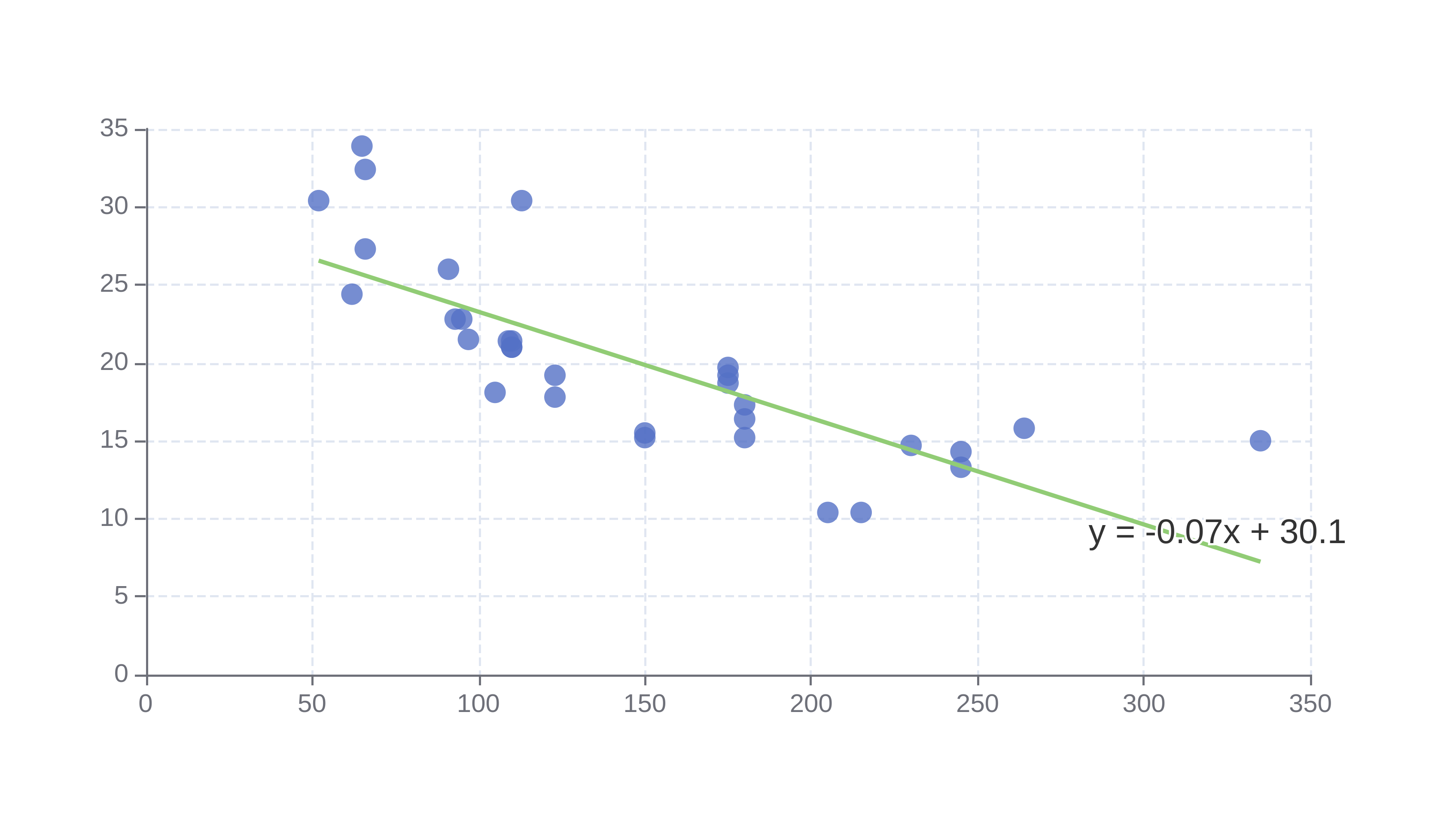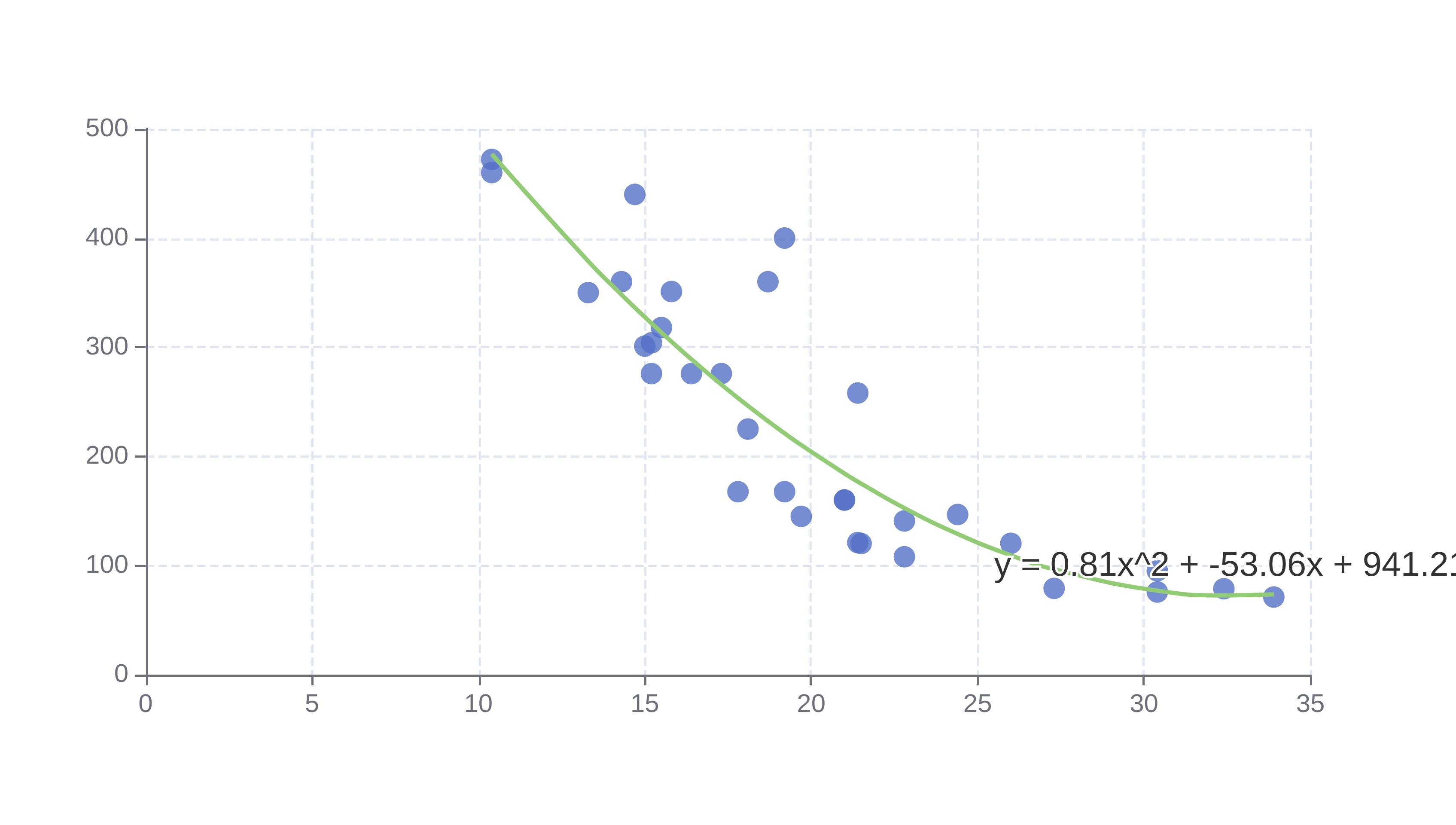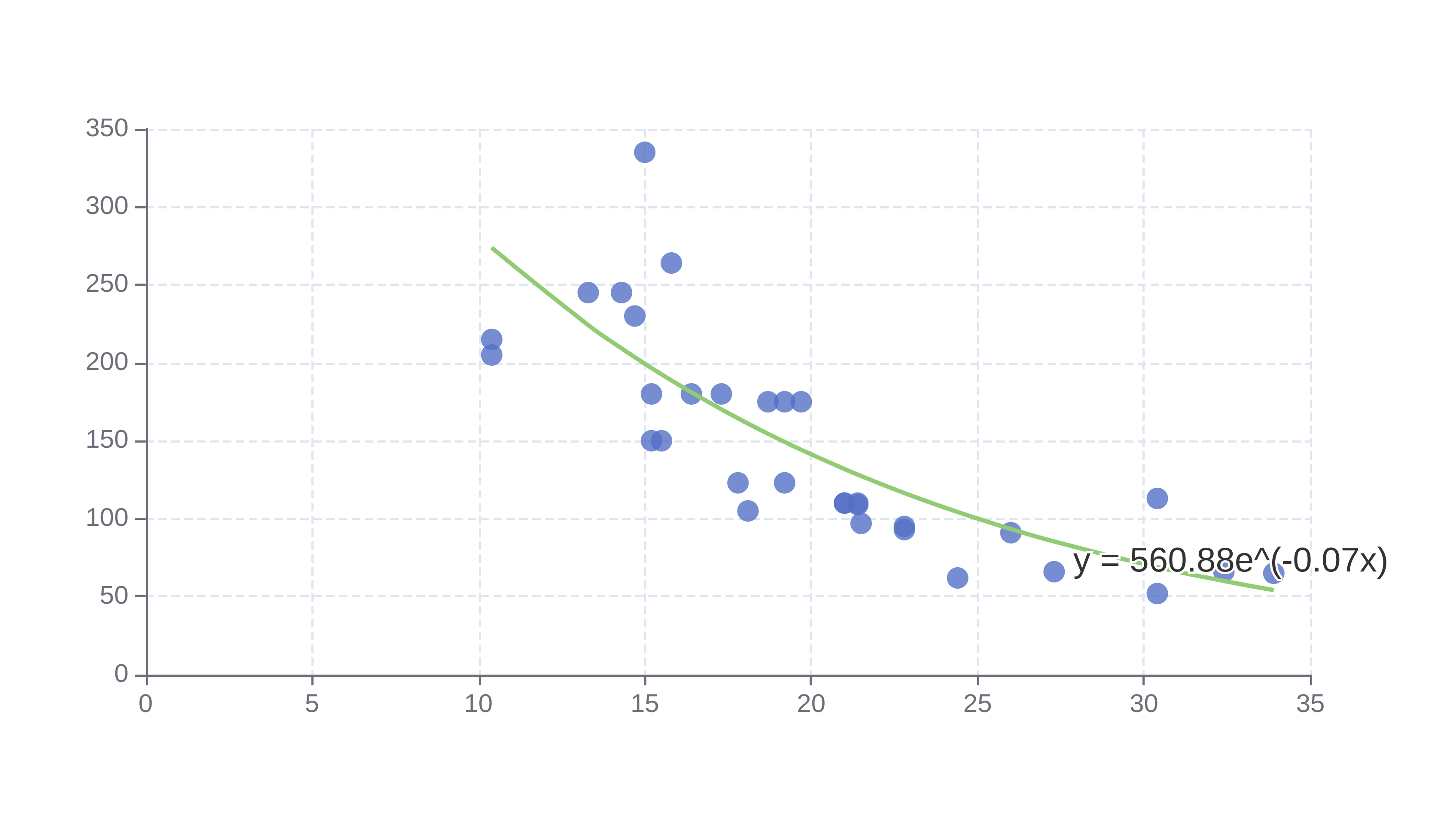# Regression Calculator

Or input your data as csv

Sharing helps us build more free tools

Regression is used to determine how one dependent variable is related to one or more independent variables.

Dependant variables change as the independent variables change. Usually, the independent variable is shown on the horizontal x-axis and the dependent variable is shown on the vertical y-axis.

For example, you could use a regression analysis to determine the relationship between the age of a car and how far it has driven. You could use the regression fit to understand how mileage changes with car age, or predict how far the car will be driven in the next year.

## When To Use Regression

Regression is a useful tool for understanding relationships between variables and forecasting.

If you understand the relationship between an independent and dependent variable then you can make changes to the independent variable to influence the dependent variable. If you understand how the independent variable will change with time, then you can use the regression fit to forecast the value of the dependent variable over time.

## Types of Regression

### Linear Regression

Linear regression fits a line of best fit to the input data points using a linear model.

Linear regression can be used to fit a straight line to the input data points using the regression line equation.Where y is the response variable, a is the slope of the line, x is the predictor variable and b is the y intercept of the fit.

A linear regression model fits the slope of the line, a, and the y intercept b.

The chart below shows a first order linear regression fit where the slope coefficient (a) is -0.07 and the y intercept (b) is 30.1.The linear regression calculator can be used to fit straight lines to input data.

#### Polynomial Regression

Linear regression equation can be extended to fit polynomials (a quadratic function) to input data points.

The general form of the linear regression equation is:Where a_n ... a_1 and b are all coefficients fit by the linear regression model.

The chart below shows a second order polynomial fit with a_2 = 0.81, a_1 = 53.06 and b = 941.2.The regression equation for the model fit above is:### Exponential Regression

The exponential regression calculator can be used to fit an exponential function to input data points.

The exponential regression equation is:Where y is the predicted value for the response variable, x is the predictor variable, a and b are fit by the exponential regression calculator.

The following chart shows an exponential regression fit with a=560.88, b=-0.07.The exponential regression fit for the chart above is: